PHY294H - Lecture 10

Suggested reading for Lecture 11: FGT pp691-706

Continuous distributions of charge

Uniform ring

Consider a thin uniform ring of charge densityand radius R. Consider that the ring is centered at the origin and lies in the x-y plane. Find the electric potential at position z on the z-axis. From this electric potential confirm the result we have before for the electric field at this point ie. Ez(z) = kQz/(R2+z2)3/2.

Solution

Break the ring up into angular segments, with the angle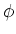going from 0 to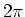as it goes around the ring. The amount of charge in a small segment of the ring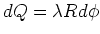. The distance from this small element of charge to the position z is r=(z2+R2)1/2. The contribution of this small element of charge to the total potential at z is kdQ/r. We find the total contribution by integrating,(1)

To find the electric field,, which yields, Ex=0=Ey and Ez as given in the problem statement.

Uniform disc

Consider a thin uniform disc of charge density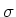and radius a. centered at the origin and lying in the x-y plane. Find the electric potential at position z on the z-axis and from this electric potential find the electric field at this point.

Solution

Break the disc up into uniform rings of charge, with each ring having width dR. The charge in each ring is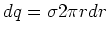. The potential due to a ring of charge is given in Eq. (1) except we need to make the substitution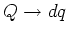. Then we need to integrate from 0 to a, that is,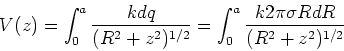(2)

Carring out the integral, we find,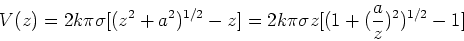(3)

At long distances, we can expand the square root to leading order, using the expansion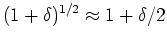. We then find,(4)

The last expression on the RHS is the potential of a point charge, which is what we would expect at long distances.

Now we look at two cases that seem strange. The infinite sheet and the infinite rod of charge. In these cases we do not take the limit of integration to infinity.

An infinite sheet of charge

Consider an infinite sheet of charge densitylying in the x-y plane. We want to find the electric potential at a position z above this sheet of charge. The electric field due to this sheet of charge is. Therefore the electric potential is,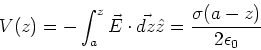(5)

Note that this diverges if we try to take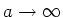. This is not surprising as we are working against a finite electric field over an infinite distance. This just means that we have to take our reference potential in this calculation to be at a finite location. In reality we shall always treat two sheets whose charges add up to zero. That way there is not problem at long distance.

An infinite rod of charge

From Gauss' law, the electric field outside an infinite uniform rod of charge densityis given by,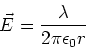(6)

The potential at position r is then,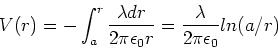(7)

Again this diverges if we take, however it is a very useful formula when considering concentric cylinders, like co-axial cables etc. In these cases we have two cylinders which have equal and opposite charges, so there is no divergence at long distances.

Sharp metal objects

Sharp metal tips are surprisingly important. From lightning rods to tunnelling tips in scanning microscopes we use sharp metals tips to control and/or enhance electric fields. Due to the fact that the electric field inside a conductor is zero, if there is no current flow, a conductor in an external electric field causes strong deformations of the electric field lines and the equipotential lines. These deformations are particularly important near sharp metal tips where the electric field is strongly enhanced. For this reason, conducting metal rods are used for lightning protection and as atomic scale probes of materials.

As a simple example, consider two metal spheres of radii r1 and r2 respectively. Place these spheres well apart so that they do not polarize each others charge distribution significantly. Now connect the two metal spheres using a conducting wire. Since the metals are conducting, and when there is no current flowing, the two spheres are at the same potential, e.g. about 500kV if we use our Van de Graaff generator. Now we demonstrate that by using the potential and the radii r1 and r2, we can make a electric field amplifier. Let the charge on sphere one be Q1 and the charge on sphere two be Q2. Then we have,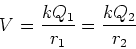(8)

The magnitude of the electric field just outside the surface of the first sphere is,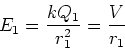(9)

while the magnitude of the electric field just outside the surface of the second sphere is,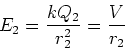(10)

It is clear that if the radii are made small, then the electric field is large. This is illustrated by using the ratio,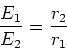(11)

If we choose r1<<r2 it is evident that the electric field ratio can be made arbitrarily large.

The strong electric field enhancement near the small metal sphere in the above example is a simple illustration of a very general effect. An important more general result is that a sharp metal object aligned along the direction of a uniform electric field has a very strong electric field enhancement at its tips. If the sharp metal object has length a and radius of curvature r at its tip, then it can be shown that the electric field enhancement at the tip is given by,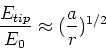(12)

In atomic microscopy, tips of radii of order 50nm are not unusual. These tips produce strong electric fields which are used for a variety of purposes in nanoengineering. For example a strong non-uniform electric field can attract atoms (through either a permanent or induced dipole moment of the atom). Atoms can then be picked up by a small metal tip and placed at a desired location on the surface. This has been demonstrated by a group at IBM Almaden reseach labs., but it is still very laborious.